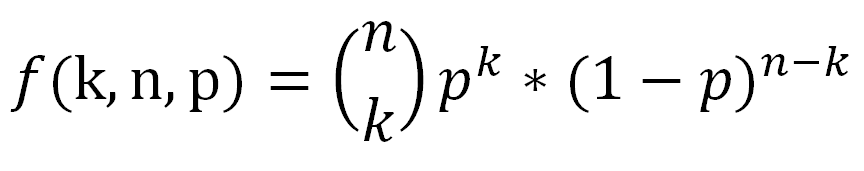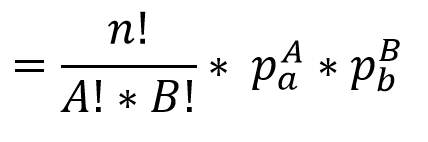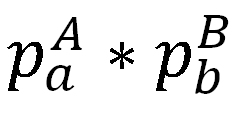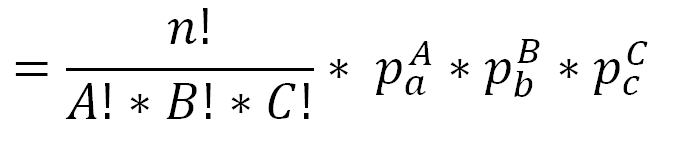# Binomial Equation – Made Easy To Remember

The binomial equation calculates the probability of getting a certain outcome after a number of discrete events.  For instance, if your team has a 60% chance of winning any single game against another team, what are the odds that they will win at least 4 out of 7 games in the championship series against that other team?

The most common equation you see for the binomial distribution isAs far as what the letters mean

• f – just means it is a function of k, n, p
• k – This is the number of successful events. e. the total number of heads in the coin flips, or the total number of outcome A out of A/B
• n – This is the total number of events. This would be all the flips, regardless of the outcome
• p – This is a decimal number between 0 and 1 inclusive representing the probability of a successful event on a single trial. For instance if you were rolling a die and it only counted if you got a 6, then p would be 1/6 = .1667

However I think the equation is easier to remember when written in a slightly different way, asHere A is the number of times outcome A occurs, so it is equal to k from the previous equation.  B is the number of times outcome B occurs, and is equal to (n-k) when there are two events.  pa is the probability that outcome A occurs and pb is the probability that outcome B occurs

## What You Already Know In The Binomial Equation

The reason I like the equation in this form is that it builds on information that you already know.  For instance, say that you have an outcome that has a 1/6 chance of occurring, like rolling a 6 on a die.  What are the odds that event will occur 10 times in a row?   Well the odds of that happening are 1/6 raised to the 10th power.  You likely already know that, and in equation form it would beNow let’s say that you have two different outcomes, A and B.   What is the probability that outcome A will occur A times in a row, and then outcome B will occur B times in a row?   This is just the probability of A, raised to the power of A times, multiplied by the probability of B, raised to the power of B times.For instance, if you wanted to calculate the odds of rolling a die 5 times in a row and getting 6 every time, and then flipping a coin 4 times in a row and getting heads every time it would just be  1/6 raised to the 5th power, multiplied by 1/2 raised to the 4th power.  That is simple enough, easy to understand, and frankly you don’t need a special equation in order to remember it.  And that is the second half of the binomial equation.The second half of the binomial equation is just   “If outcome A had to occur all the times in a row, then outcome B all the times in a row, what is the probability that string of outcomes would occur?”

## 1st Half Of Binomial Equation

Ok, that is the second half of the equation with the exponentials, what about the first half of the equation?This is the combination equation.  There are a lot of interesting things to know about the combination equation, and those are discussed in this blog post on combinations and permutations.  For our purposes right now, the best way to think of this part of the equation is as such:   It is the number of different orders of ways you can do outcome A and outcome B.   i.e. it is the number of different tries you get at making your desired outcome happen.

As an example, let’s say that outcome A was rolling a 6 on a die, and outcome B was flipping a heads on a coin, and you needed to do that two times each.   If you had to roll a 6 twice in a row, and flip the coin twice in a row the probability would beAs we showed before.   However now let me tell you that you get to try again, however many times you can reorder the events.  For instance, the first trial was

• Roll-Roll-Flip-Flip

So that is one order.  But you can also try again

• Roll-Flip-Roll-Flip

And

• Roll-Flip-Flip-Roll

And so on.  There are in fact 6 unique orderings of 2 rolls and 2 flips.   This can be calculated by 4! / (2!*2!)  =   24 / (2*2) = 6       here the 4! Is because the total number of events is 4  (2+2)   and each of the two factorial is because there are two trials of event A, and two trials of event B.

The 6 unique orders of rolls and flips, as A’s and B’s are

• A-A-B-B
• A-B-A-B
• A-B-B-A
• B-A-B-A
• B-B-A-A
• B-A-A-B

So the first half of the equationTells you the number of times you get to try to get a specific outcome, and the second half of the equationTells you the probability of that outcome if done a single time.   Combined they give the full probability of an outcome## Example Problem Solved

Let’s take it back to the starting example.  Your team has a 60% chance of winning.  What are the odds they will win exactly 4 games in 7 ?

• Outcome A is that your team wins. This occurs 4 times, and a has a probability of .6
• Outcome B is that the other team wins. This occurs 3 times and has a probability of .4.

There are 7 events in all.  The equation becomesSo the odds of your team winning exactly 4 games are 29.03%.

## What Are The Odds Of At Least 4 Games?

But wait, the real question we asked was the odds of winning at least 4 games, not exactly 4 games.  Your team could win 5 games, or 6 games, or all 7.  That means we have to calculate the answer again for each of those possible number of wins.  That calculation is done belowThe end result is that if your team has a 60% chance of winning any single game, you expect that they have a 71.02% chance of winning at least 4 games in a 7 game series.

If you increased the number of games, and went best 5 of 9, or best 6 of 11 the likelihood of the team with the higher winning percentage winning the series increases.

## Multinomial Equation

There is another reason why I like the binomial equation rewritten the way we did it.  That is because it is easy to expand that equation from a Binomial equation to a Multinomial equation.  I.e. it is easy to change from 2 outcomes to 3 or more outcomes.

What would be a multinomial event?  Well for instance, instead of having two teams play, think of three people racing in a track meet.  So instead of calculating the odds of one team winning 4 games out of 7, what are the odds that person A will win 4 races out of 7, person B will win 2 races out of 7, and person C will win one?

Here assume the odds of person A, B, and C winning any given race are 50%, 40%, and 10% respectively.

Getting the equation for 3 possible outcomes from the 2 possible outcome equation is almost trivial.  It isOnce again, the second half of the equation is the probability that that specific chain of outcomes will occur, and the first half of the equation is the number of different paths you can take to get there.

For this specific racing problem the equation becomes